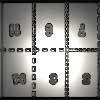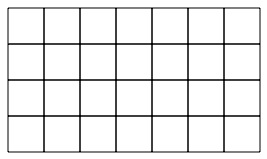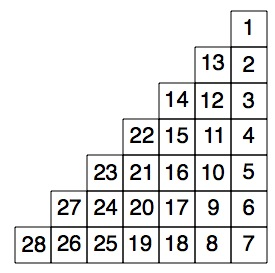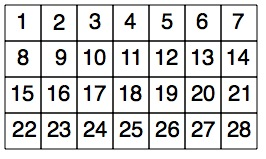#### You may also like### Exploring Wild & Wonderful Number Patterns

EWWNP means Exploring Wild and Wonderful Number Patterns Created by Yourself! Investigate what happens if we create number patterns using some simple rules.### Magazines

Let's suppose that you are going to have a magazine which has 16 pages of A5 size. Can you find some different ways to make these pages? Investigate the pattern for each if you number the pages.### Pebbles

Place four pebbles on the sand in the form of a square. Keep adding as few pebbles as necessary to double the area. How many extra pebbles are added each time?

# Month Mania

##### Age 5 to 11Challenge Level

When it's the end of January and we need to turn over a page of our calendar, the next page may look a bit like this:Of course, it probably has a good picture on the page as well, perhaps of a scene from a T.V. show or an animal from your favourite story book.

If we ignore the edge squares which tell you what day of the week it is, then we have a rectangle with twenty eight squares in it like this:-Well, how about going a bit wild and designing a new shape for these twenty eight squares in which the numbers follow on in some way that is not too difficult to follow? For example, you could put the twenty eight squares into a triangle and decide to go in an up-and-down order like this:-What other wonderful designs can you come up with?

You can then go further by looking at the way the numbers are arranged and see if you can spot any patterns. For example in the first usual way:-you may notice things like:

1. If you take any two by two square (like the one in at the top left-hand corner with $1$, $2$, $8$ and $9$ in it), then the opposite corners of that square add up to $10$. Then, if you take the next two by two square along [with $3$, $4$, $10$ and $11$ in] then the opposite corners add up to $14$ and so on.

2. Going down any column the numbers increase by $7$.

Do let us know what you discover in the designs you have created. Can you explain why the patterns occur?

Then ask "I wonder what would happen if ...?''